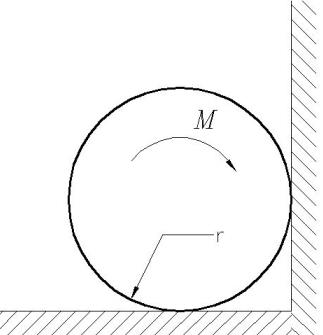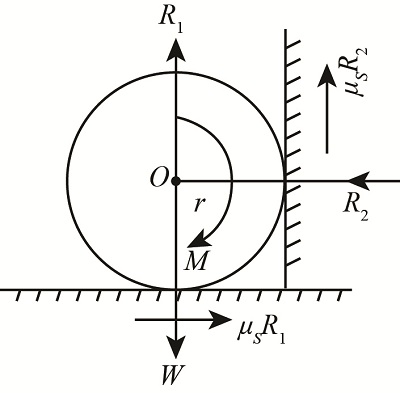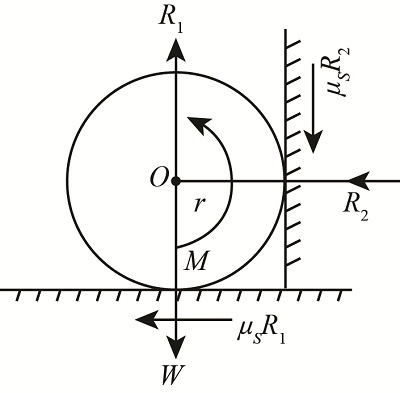# Find the magnitude M of the maximum moment that may be applied on the cylinder of mass m and...

## Question:

Find the magnitude M of the maximum moment that may be applied on the cylinder of mass m and radius r shown in the figure without inducing sliding. Let the static coefficient of friction be mu s on both frictional interfaces.

•How would the answer to this problem change if the sense of the moment is reversed?

## Friction

Friction is the term used in the engineering for the different application like friction can retard the motion of the particle, or the resistance can support the action it will depend upon the type of use.

## Answer and Explanation:

Given data

• Magnitude of the maximum moment is {eq}M {/eq}
• Mass of the cylinder is {eq}m . {/eq}
• Radius is {eq}r . {/eq}
• Static friction is {eq}{\mu _s} {/eq}

Free body diagram of the cylinder isExpression for the weight is

{eq}W = mg {/eq}

Here, the weight is {eq}W {/eq} , and the gravity is {eq}g {/eq}

Expression for the vertical reaction is

{eq}\begin{align*} {R_1} &= W\\ &= mg \end{align*} {/eq}

Here , and the normal reaction is {eq}{R_1} {/eq}

Expression for the horizontal reaction is

{eq}\begin{align*} {R_2} &= {\mu _s}{R_1}\\ &= {\mu _s}mg \end{align*} {/eq}

Expression for the moment about O is

{eq}M = {\mu _s}{R_1} \times r + {\mu _s}{R_2} \times r {/eq}

Substitute the given value in the above expression

{eq}\begin{align*} M &= {\mu _s} \times mg \times r + {\mu _s}\left( {{\mu _s}mg} \right) \times r\\ &= {\mu _s}mgr\left( {1 + {\mu _s}} \right) \end{align*} {/eq}

Thus, the magnitude of the moment is {eq}{\mu _s}mgr\left( {1 + {\mu _s}} \right) {/eq}

Free body diagram of the cylinder when moment is reversed.If direction of the moment is reversed then the direction of the frictional forces are changes but the magnitude of the moment is remains same.

#### Learn more about this topic:What is Torque? - Definition, Equation & Calculation

from Holt McDougal Physics: Online Textbook Help

Chapter 4 / Lesson 11
779K